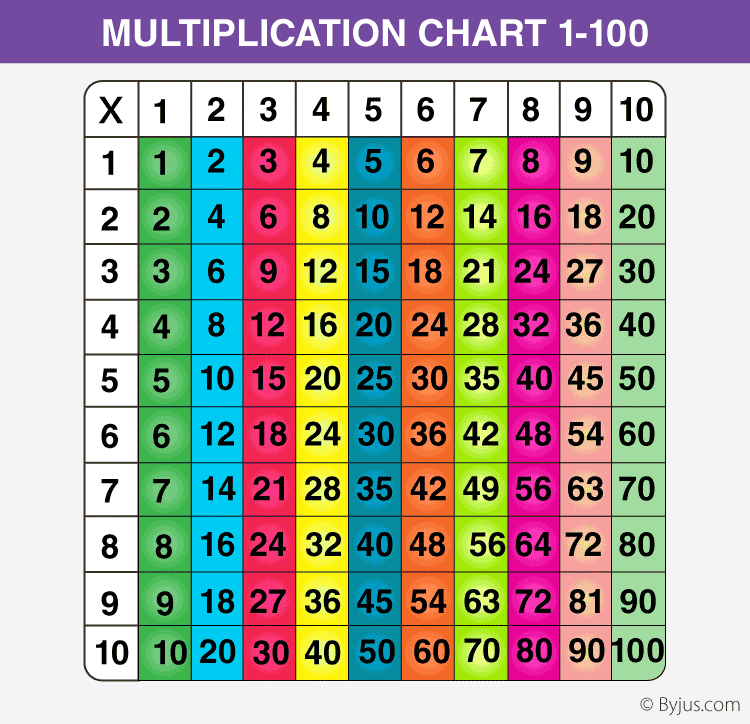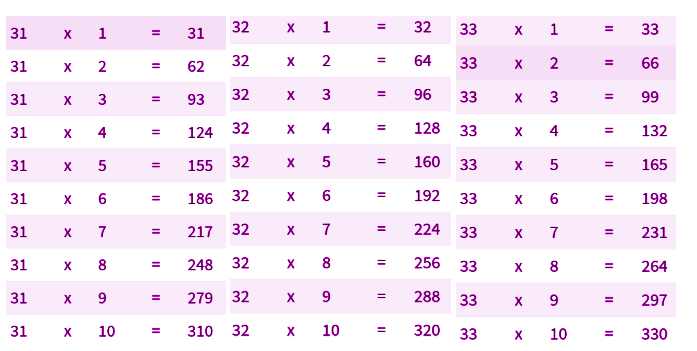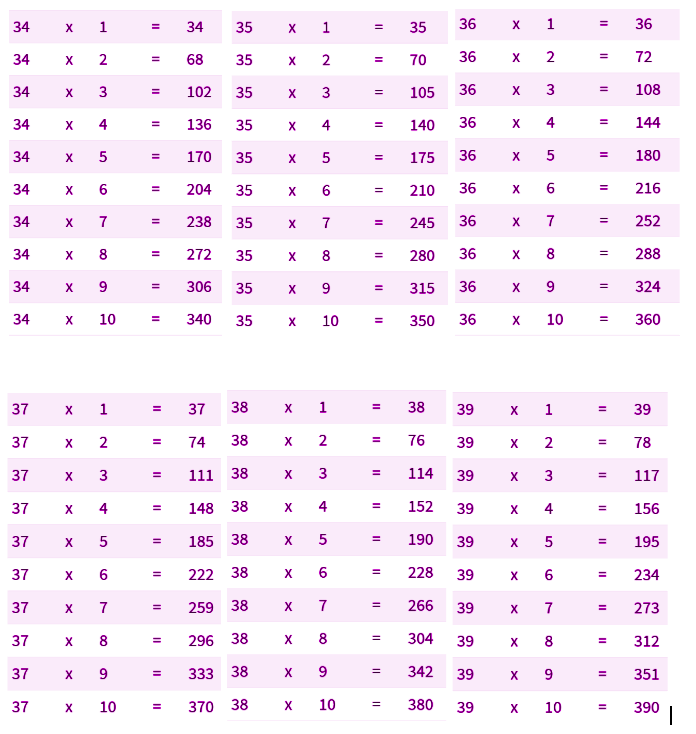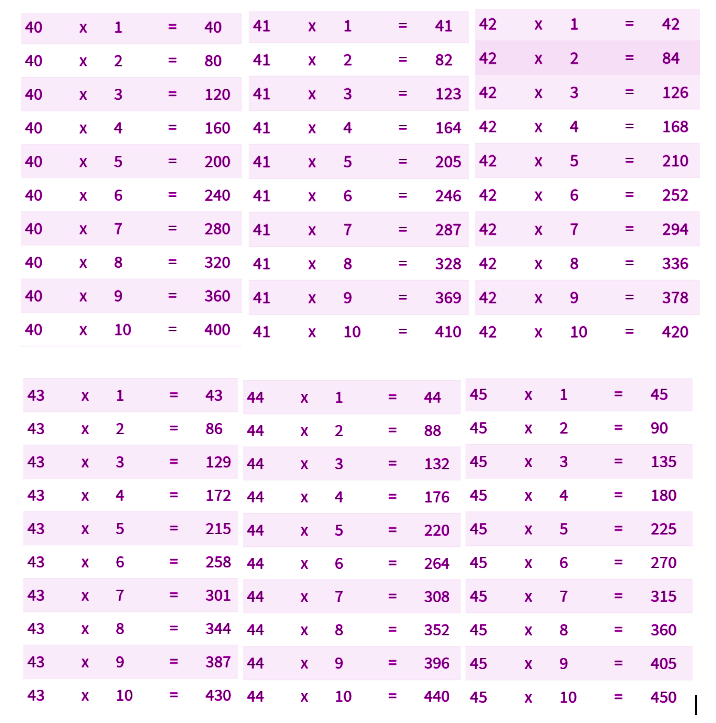# Tables 1 to 100

You’ll find multiplication tables 1 to 100 on this web page. Learn all the tables from 1 to 30 by reading them aloud from the charts given in this page. The basic numeracy skill improves by learning the maths tables from 1 to 20. It would be easy to solve any type of mathematical problem even if you learn the tables from 2 to 10 properly. We have presented all the tables in a colourful table or chart method to make it more interesting to learn. Also, BYJU’S provides table in PDF format, which students can download and learn offline as well.

## Tables from 1 to 100

Memorizing the tables 1 to 30 first will help you in learning rest of the 1 to 100 tables and also in solving any kind of problem and in using all kinds of mathematical operations like Addition, Subtraction, Division and Subtraction. For example: Instead of adding 2 + 2 + 2 + 2 + 2 + 2 + 2 + 2 + 2 + 2, 10 times, you can calculate it fast if you know how much is 10 times 2 i.e 20. Not only memorizing tables helps in making calculations easy but fast too. These multiplication tables from 2 to 30 will help you in scoring good marks by making it easier to solve the problems fast.## Tables From 2 to 30

Find the links to tables from 1 to 30. These tables are given in table and chart form to make it easier for students to learn it quickly.

## Multiplication Tables 1 to 100

We need a strong base of multiplication table in maths to solve any kind of Mathematical problem. You can learn these 1 to 100 tables, which are given in a table form here. You can also use the multiplication table calculator to find any of the tables you need to learn. Here’s the link for the same: